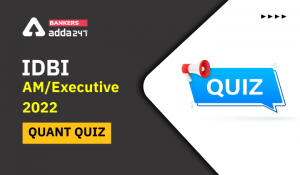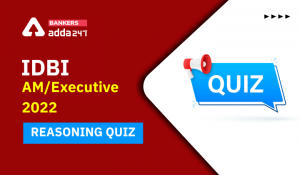Latest Banking jobs   »   Reasoning Ability Quiz For SBI PO,...

# Reasoning Ability Quiz For SBI PO, Clerk Prelims 2021- 1st May

Directions (1-5): Study the set of numbers given below and answer the questions which follow:

924 643 867 758 568

Q1. If in each number, the first and the last digits are interchanged, then which of the following will be the third highest number?
(a) 924
(b) 643
(c) 867
(d) 758
(e) 568

Q2. If in each number, all the three digits are arranged in the descending order, then which of the following will be the third lowest number?
(a) 643
(b) 568
(c) 924
(d) 867
(e) 758

Q3.What will be the resultant, if the second digit of the lowest number is subtracted from the first digit of the largest number?
(a) 1
(b) 2
(c) 3
(d) 4
(e) 0

Q4. If in each number, the second and the first digits are interchanged, then which will be the highest number?
(a) 758
(b) 867
(c) 643
(d) 924
(e) 568

Q5. If one is subtracted from the first digit of each of the numbers and then the first and the third digits are interchanged, then which of the following will be the lowest?
(a) 643
(b) 924
(c) 758
(d) 867
(e) 568

Directions (6-10): Study the following arrangement carefully and answer the questions given below:

4 2 3 5 1 4 9 4 1 5 2 8 3 9 1 4 1 2 5 7 3 5 9 6 4 2 5 1 8 5 2 3 4

Q6. How many 3s are there in the above arrangement, each of which is immediately preceded by a digit which has a numerical value of more than five?
(a) None
(b) One
(c) Two
(d) Three
(e) More than three

Q7. How many such 1s are there in the above arrangement each of which is immediately preceded by an odd digit and immediately followed by an even digit?
(a) None
(b) One
(c) Two
(d) Three
(e) More than three

Q8. What is the sum of the digit which is 12th from the left end and 16th from the right end?
(a) 10
(b) 15
(c) 7
(d) 6
(e) 5

Q9. Which of the following is 7th to the right of the 20th from the left end of the above arrangement?
(a) 8
(b) 5
(c) 2
(d) 4
(e) 1

Q10. If all the odd digits are deleted (dropped) from the above arrangements, then which of the following will be ninth from the right end of the arrangement?
(a) None
(b) 8
(c) 6
(d) 4
(e) 2

Directions (11-15): These questions are based on the following six numbers.

312 876 156 275 734 954

Q11. If we add 2 to the second digit of each number and subtract 1 from the first digit of each number, then which of the given will become the lowest number?
(a) 312
(b) 876
(c) 156
(d) 275
(e) None of these

Q12. If all the digits in each of the numbers are written in ascending order within the number, then which of the given number will become the second highest?
(a) 312
(b) 954
(c) 156
(d) 275
(e) None of these

Q13. If the first two digits of each number are interchanged, then which of the following numbers will become the highest number?
(a) 721
(b) 876
(c) 937
(d) 387
(e) 275

Q14. In each number, if we subtracted 1 in first digit and added 1 in last digit, then which among these number will be 2nd lowest number?
(a) 734
(b) 876
(c) 954
(d) 275
(e) None of these

Q15.If we replace all the odd digits in each number with zero, then which of the following number will become the lowest?
(a) 312
(b) 876
(c) 156
(d) 275
(e) 734

Practice More Questions of Reasoning for Competitive Exams:

###### Study Plan for SBI Clerk/PO 2021: Prelims Exam

Solutions

S1. Ans.(c)
Sol. 429 346 768 857 865

S2. Ans.(e)
Sol. 942 643 876 875 865

S3. Ans.(c)
Sol. lowest number- 568
Largest number- 924

S4. Ans.(b)
Sol. 294 463 687 578 658

S5. Ans.(a)
Sol. 428 345 767 856 864

S6. Ans.(c)
Sol. 83, 73

S7. Ans.(d)
Sol. 514, 914, 518

S8. Ans.(a)
Sol. 12th from the left end= 8
16th from the right end= 2
Sum= 10

S9. Ans.(b)
Sol. 7th to the right of the 20th from the left end= 27th from the left end= 5

S10. Ans.(b)

S11.Ans.(c)
Sol. 232, 796, 076, 195, 654, 874

S12.Ans.(b)
Sol. 123, 678, 156, 257, 347, 459

S13.Ans.(b)
Sol. 132, 786, 516, 725, 374, 594

S14.Ans.(d)
Sol. 213, 777, 057, 176, 635, 855

S15.Ans.(a)
Sol. 002, 806, 006, 200, 004, 004

#### Congratulations!Incorrect details? Fill the form again here

•Reasoning Ability Quiz For IBPS RRB PO C...
•Reasoning Ability Quiz For IBPS Clerk Pr...
•Quantitative Aptitude Quiz For IDBI AM/E...
•Quantitative Aptitude Quiz For IDBI AM/E...
•Reasoning Ability Quiz For IBPS RRB PO P...
•Reasoning Ability Quiz For IDBI AM/Execu...